### Home > GC > Chapter 9 > Lesson 9.2.3 > Problem9-81

9-81.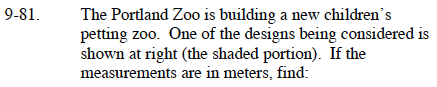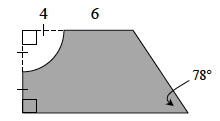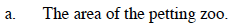Find the area of the pen by first finding the area of the trapezoid. Notice the congruent segments in the right hand side. Then subtract the area of the quarter of the circle.

Draw a height to make a right triangle. The height is 8 m. Use trigonometry to find the unknown side lengths.
Remember, the formula for the area of a circle is A = r²π.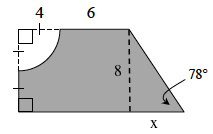$\text{tan}(78\degree)=\frac{8}{x}$

$x\cdot\text{tan}(78\degree)= 8$

$x=\frac{8}{\text{ tan}(78\degree)}$

$\text{Area }=\left[\frac{1}{2}(\text{base}_{1}+\text{base}_{2})\text{height}\right]-[\text{radius}^{2}\pi\div 4]$

$\left[\frac{1}{2}((4+6)+\left ( 4+6+\frac{8}{\text{tan}(78\degree)} \right ))(4+4)\right]-[4^{2}\pi\div 4]$

$\frac{1}{2}\left(20+\frac{8}{\text{tan}(78\degree)}\right)8-(16\pi\div 4)$

$80+\frac{32}{\text{tan}(78\degree)}-4\pi$

≈ 74.24 meters2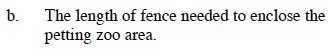The formula for the circumference of a circle is C = 2πr. Remember it is only a quarter of a circle.

Meters of fencing need is ≈ 36.2 meters